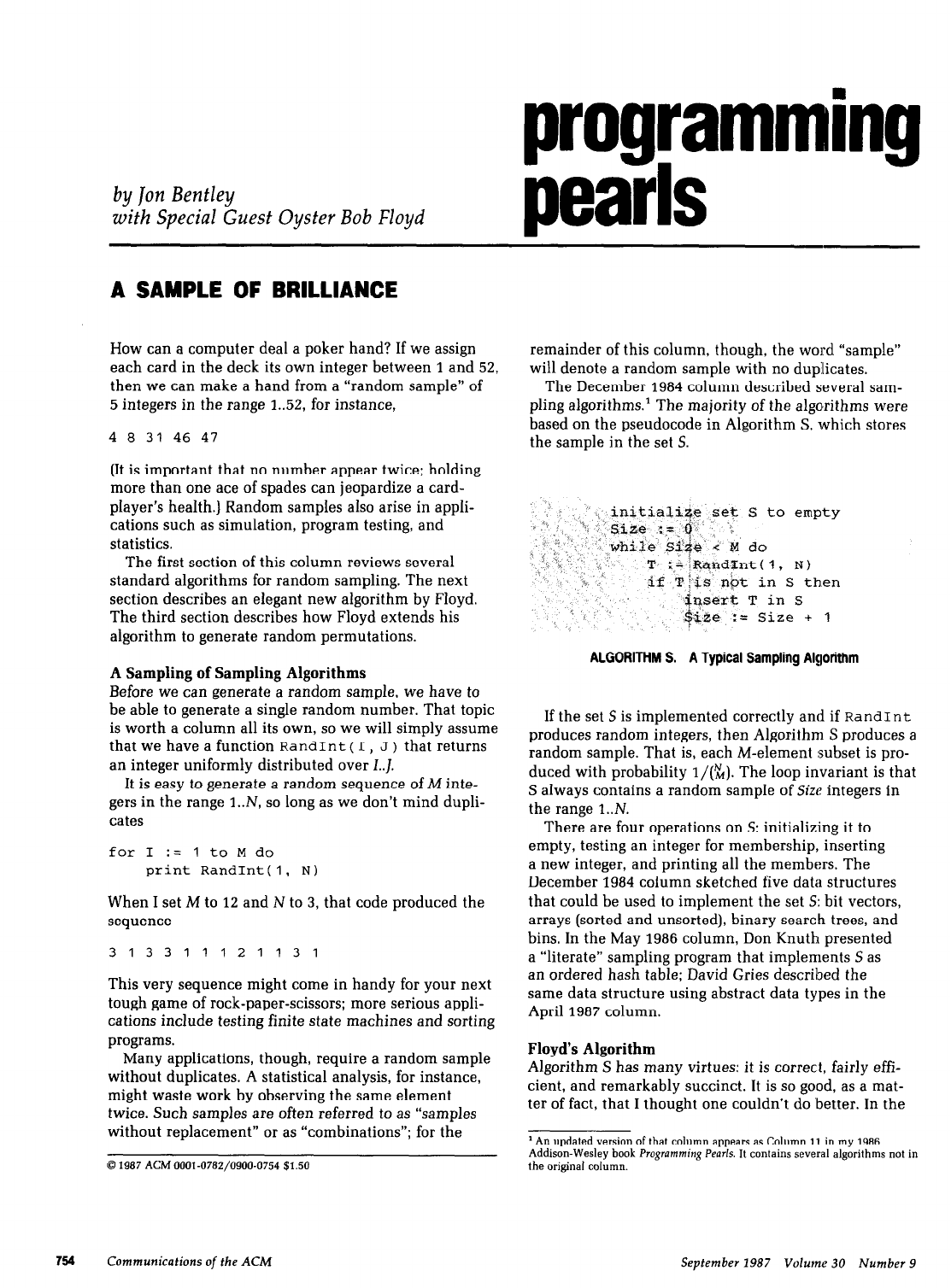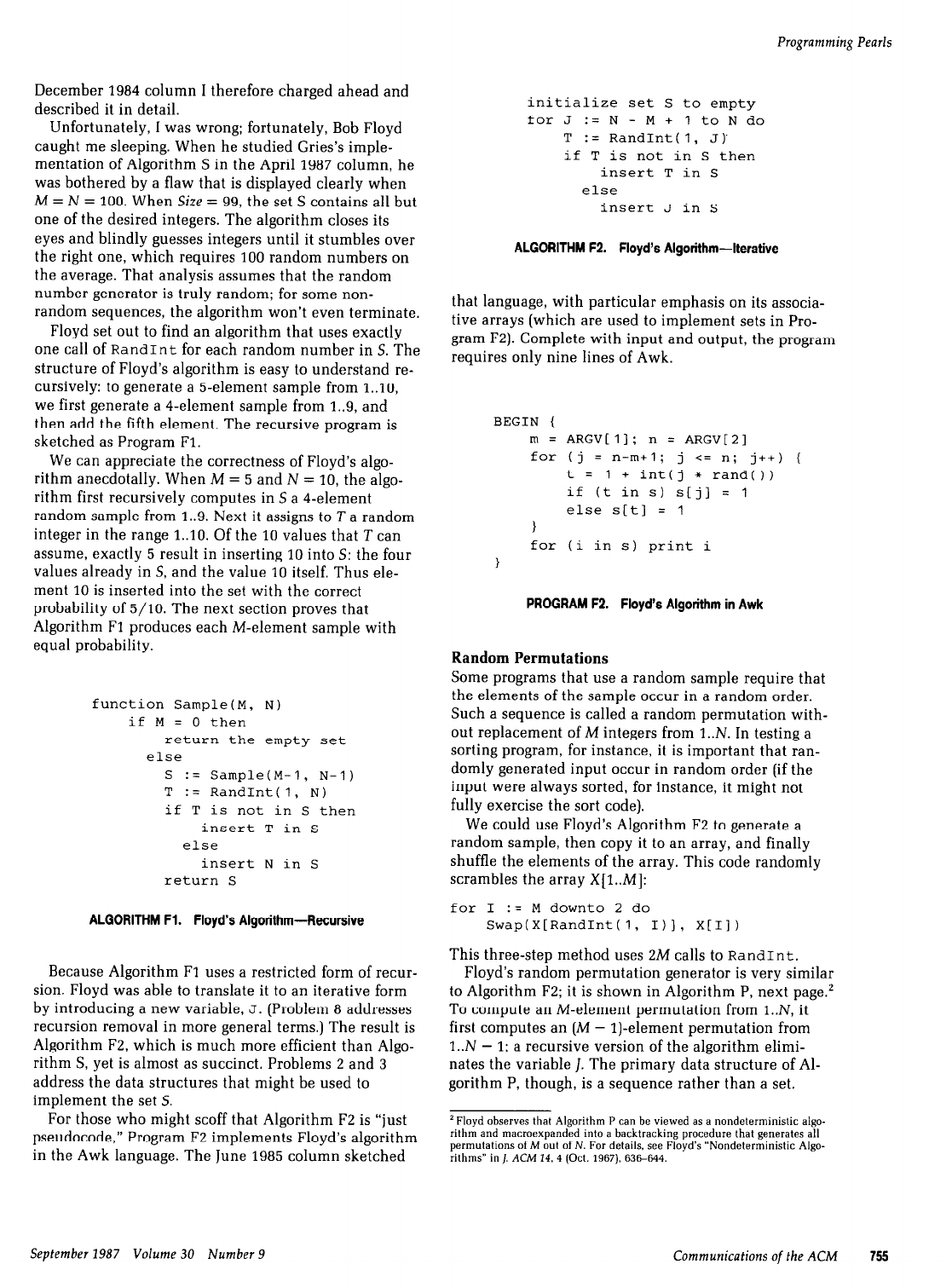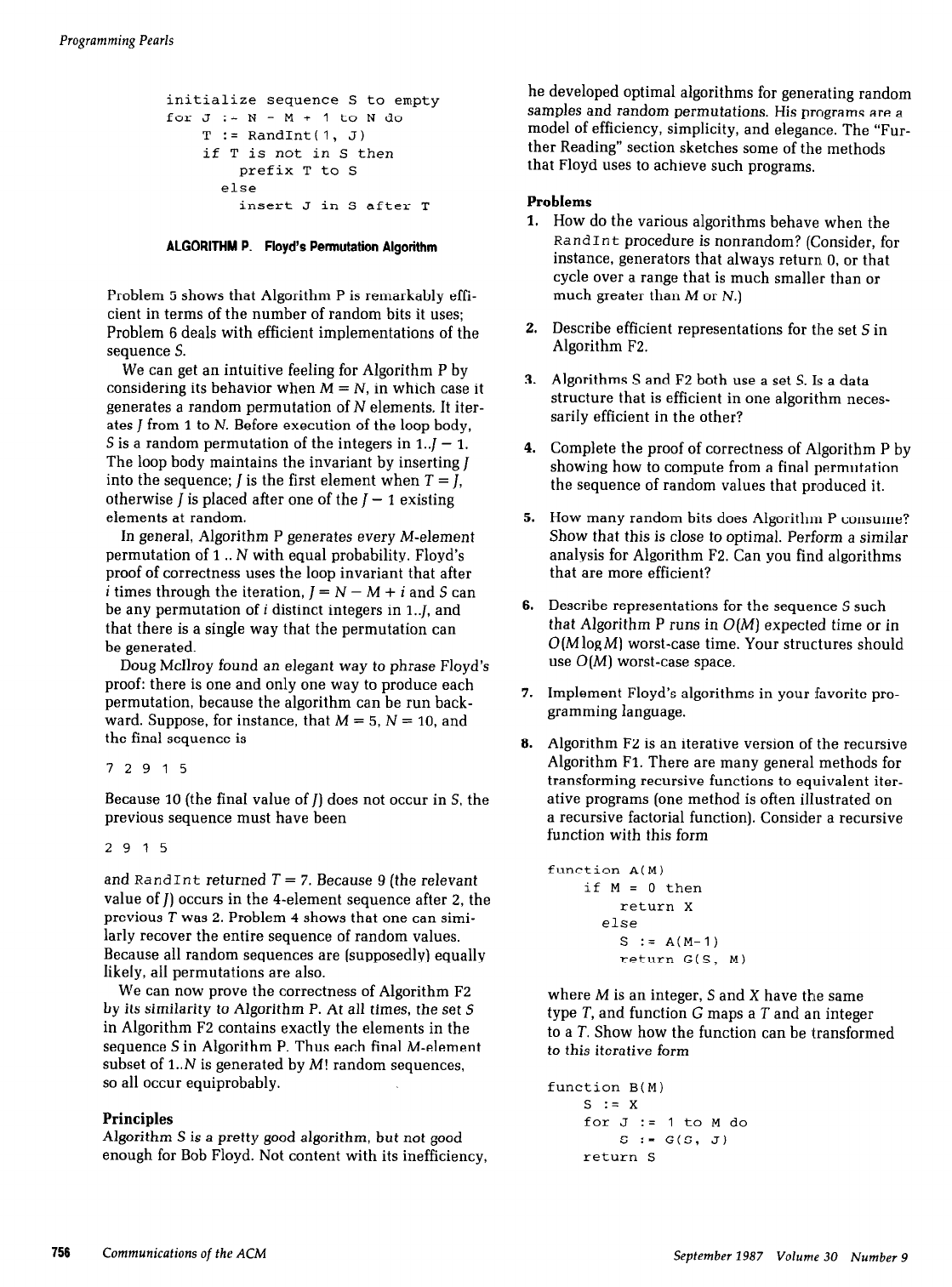Jon Louis Bentley is an American computer scientist. He received a ...
Robert Floyd was an eminent American Computer Scientist who receive...
#### Random Number Generators Random numbers are a very interest...
If you want to appreciate just how hard it can be to generate rando...
Here is the 1986 column Bentley is talking about: [Literate Program...
Let me attempt to simplify Floyd's algorithm by first teasing out s...
This is the modern version of the Fisher-Yates shuffling algorithm,...programming
by
jon
Bentley
with Special Guest Oyster Bob Floyd
pearls
A SAMPLE OF BRILLIANCE
How can a computer deal a poker hand? If we assign
each card in the deck its own integer between 1 and 52,
then we can make a hand from a “random sample” of
5 integers in the range 1..52, for instance,
4 a 31 46 47
(It is important that no number appear twice; holding
more than one ace of spades can jeopardize a card-
player’s health.) Random samples also arise in appli-
cations such as simulation, program testing, and
statistics.
The first section of this column reviews several
standard algorithms for random sampling. The next
section describes an elegant new algorithm by Floyd.
The third section describes how Floyd extends his
algorithm to generate random permutations.
A Sampling of Sampling Algorithms
Before we can generate a random sample, we have to
be able to generate a single random number. That topic
is worth a column all its own, so we will simply assume
that we have a function
RandInt
( I,
J
) that returns
an integer uniformly distributed over I..J
It is easy to generate a random sequence of
M
inte-
gers in the range l..N, so long as we don’t mind dupli-
cates
for I :=
1
to M do
print
RandInt(1, N)
When I set
M
to 12 and N to 3, that code produced the
sequence
313311121131
This very sequence might come in handy for your next
tough game of rock-paper-scissors; more serious appli-
cations include testing finite state machines and sorting
programs.
Many applications, though, require a random sample
without duplicates. A statistical analysis, for instance,
might waste work by observing the same element
twice. Such samples are often referred to as “samples
without replacement” or as “combinations”; for the
0 1987 ACM OOOl-0782/0900-0754 \$1.50
remainder of this column, though, the word “sample”
will denote a random sample with no duplicates.
The December 1984 column described several sam-
pling algorithms.’ The majority of the algorithms were
based on the pseudocode in Algorithm S, which stores
the sample in the set S.
en
ALGORITHM S. A Typical Sampling Algorithm
If the set S is implemented correctly and if
RandInt
produces random integers, then Algorithm S produces a
random sample. That is, each M-element subset is pro-
duced with probability I/(%). The loop invariant is that
S always contains a random sample of Size: integers in
the range l..N.
There are four operations on S: initializing it to
empty, testing an integer for membership, inserting
a new integer, and printing all the members. The
December 1984 column sketched five data structures
that could be used to implement the set S: bit vectors,
arrays (sorted and unsorted), binary search trees, and
bins. In the May
1986
column, Don Knuth presented
a “literate” sampling program that implements S as
an ordered hash table; David Gries described the
same data structure using abstract data types in the
April 1987 column.
Floyd’s Algorithm
Algorithm S has many virtues: it is correct, fairly effi-
cient, and remarkably succinct. It is so good, as a mat-
ter of fact, that I thought one couldn’t do better. In the
An updated version of that column appears as Column 11 in my 1986
Programming Pearls.
It contains several algorithms not in
the original column.
754
Communications of
the ACM
September 1987 Volume 30 Number 9Programming
Pearls
December 1984 column I therefore charged ahead and
described it in detail.
Unfortunately, I was wrong; fortunately, Bob Floyd
caught me sleeping. When he studied Gries’s imple-
mentation of Algorithm S in the April 1987 column, he
was bothered by a flaw that is displayed clearly when
M = N = 100. When Size = 99, the set S contains all but
one of the desired integers. The algorithm closes its
eyes and blindly guesses integers until it stumbles over
the right one, which requires 100 random numbers on
the average. That analysis assumes that the random
number generator is truly random; for some non-
random sequences, the algorithm won’t even terminate.
Floyd set out to find an algorithm that uses exactly
one call of
RandInt
for each random number in S. The
structure of Floyd’s algorithm is easy to understand re-
cursively: to generate a 5-element sample from l..lO,
we first generate a 4-element sample from 1..9, and
then add the fifth element. The recursive program is
sketched as Program Fl.
We can appreciate the correctness of Floyd’s algo-
rithm anecdotally. When M = 5 and N = 10, the algo-
rithm first recursively computes in S a 4-element
random sample from 1..9. Next it assigns to T a random
integer in the range l..lO. Of the 10 values that T can
assume, exactly 5 result in inserting 10 into S: the four
values already in S, and the value 10 itself. Thus ele-
ment 10 is inserted into the set with the correct
probability of 5/10. The next section proves that
Algorithm Fl produces each M-element sample with
equal probability.
function SampleCM, N)
if M = 0 then
return the empty set
else
S
:= SampleCM-I,
N-l)
T := RandInt(1, N)
if T is not in S then
insert T in S
else
insert N in S
return S
ALGORITHM Fl. Floyd’s Algorithm-Recursive
Because Algorithm Fl uses a restricted form of recur-
sion. Floyd was able to translate it to an iterative form
by introducing a new variable,
J.
recursion removal in more general terms.) The result is
Algorithm F2, which is much more efficient than Algo-
rithm S, yet is almost as succinct. Problems 2 and 3
address the data structures that might be used to
implement the set S.
For those who might scoff that Algorithm F2 is “just
pseudocode,” Program F2 implements Floyd’s algorithm
in the Awk language. The June 1985 column sketched
initialize set S to empty
for J := N - M +
1
to N do
T
:= RandInt(1, J)
if T is not in S then
insert T in S
else
insert J in S
ALGORITHM F2. Floyd’s Algorithm-Iterative
that language, with particular emphasis on its associa-
tive arrays (which are used to implement sets in Pro-
gram F2). Complete with input and output, the program
requires only nine lines of Awk.
BEGIN {
m = ARGVLII; n = ARGV121
for Cj = n-m+l; j i= n; j++) {
t =
1
+ int(j * randO)
if (t in s) s[jl =
1
else s[tl =
1
for (i in s) print i
PROGRAM F2.
Floyd’s Algorithm in Awk
Random Permutations
Some programs that use a random sample require that
the elements of the sample occur in a random order.
Such a sequence is called a random permutation with-
out replacement of M integers from l..N. In testing a
sorting program, for instance, it is important that ran-
domly generated input occur in random order (if the
input were always sorted, for instance, it might not
fully exercise the sort code).
We could use Floyd’s Algorithm F2 to generate a
random sample, then copy it to an array, and finally
shuffle the elements of the array. This code randomly
scrambles the array X[l..M]:
for I
:= M downto 2 do
Swap(X[RandInt(l, I)], X[Il)
This three-step method uses 2M calls to
RandInt.
Floyd’s random permutation generator is very similar
to Algorithm F2; it is shown in Algorithm P, next page.’
To compute an M-element permutation from l..N, it
first computes an (M - I)-element permutation from
l..N - 1; a recursive version of the algorithm ehmi-
nates the variable I. The primary data structure of Al-
gorithm P, though, is a sequence rather than a set.
‘Flovd observes that Aleorithm P can be viewed as a nondeterministic aleo-
rithm and macroexpand~d into a backtracking procedure that generates aTI
permutations of M out of N. For details, see Floyd’s “Nondeterministic Algo-
rithms” in 1.
ACM 14. 4
(Oct. 1967).
636-644.
September 1987 Volume 30 Number 9
Communications
of
the ACM
755Programming Pearls
initialize sequence S to empty
for J
:= N - M +
1
to N do
T := RandInt(1, J)
if T is
not in
S then
prefix T to S
else
insert J in S after T
ALGORITHM P. Floyd’s Permutation Algorithm
Problem 5 shows that Algorithm P is remarkably effi-
cient in terms of the number of random bits it uses;
Problem 6 deals with efficient implementations of the
sequence S.
We can get an intuitive feeling for Algorithm P by
considering its behavior when M = N, in which case it
generates a random permutation of N elements. It iter-
ates J from
1
to N. Before execution of the loop body,
S is a random permutation of the integers in
1.J
- 1.
The loop body maintains the invariant by inserting J
into the sequence; 1 is the first element when T = J,
otherwise ] is placed after one of the J - 1 existing
elements at random.
In general, Algorithm P generates every M-element
permutation of
1
. . N with equal probability. Floyd’s
proof of correctness uses the loop invariant that after
i times through the iteration, J = N - M + i and S can
be any permutation of i distinct integers in
l..J,
and
that there is a single way that the permutation can
be generated.
Doug McIlroy found an elegant way to phrase Floyd’s
proof: there is one and only one way to produce each
permutation, because the algorithm can be run back-
ward. Suppose, for instance, that
M
= 5, N =
10,
and
the final sequence is
72915
Because
10
(the final value of J) does not occur in S, the
previous sequence must have been
2915
and
RandInt
returned
T
= 7. Because 9 (the relevant
value of 1) occurs in the J-element sequence after 2, the
previous T was 2. Problem 4 shows that one can simi-
larly recover the entire sequence of random values.
Because all random sequences are (supposedly) equally
likely, all permutations are also.
We can now prove the correctness of Algorithm F2
by its similarity to Algorithm P. At all times, the set S
in Algorithm F2 contains exactly the elements in the
sequence S in Algorithm P. Thus each final M-element
subset of l..N is generated by M! random sequences,
so all occur equiprobably.
Principles
Algorithm S is a pretty good algorithm, but not good
enough for Bob Floyd. Not content with its inefficiency,
he developed optimal algorithms for generating random
samples and random permutations. His programs are a
model of efficiency, simplicity, and elegance. The “Fur-
ther Reading” section sketches some of the methods
that Floyd uses to achieve such programs.
Problems
1.
2.
3.
4.
5.
6.
7.
8.
How do the various algorithms behave when the
RandInt
procedure is nonrandom? (Consider, for
instance, generators that always return 0, or that
cycle over a range that is much smaller than or
much greater than
M or N.)
Describe efficient representations for the set S in
Algorithm F2.
Algorithms S and F2 both use a set S. Is a data
structure that is efficient in one algorithm neces-
sarily efficient in the other?
Complete the proof of correctness of Algorithm P by
showing how to compute from a final permutation
the sequence of random values that produced it.
How many random bits does Algorithm P consume?
Show that this is close to optimal. Perform a similar
analysis for Algorithm F2. Can you find algorithms
that are more efficient?
Describe representations for the sequence S such
that Algorithm P runs in
O(M)
expected time or in
O(M
log
M)
use
O(M)
worst-case space.
Implement Floyd’s algorithms in your :favorite pro-
gramming language.
Algorithm F2 is an iterative version of the recursive
Algorithm
Fl.
There are many general methods for
transforming recursive functions to equivalent iter-
ative programs (one method is often illustrated on
a recursive factorial function). Consider a recursive
function with this form
function A(M)
if M = 0 then
return X
else
S := ACM-I)
return G(S, M)
where
M
is an integer, S and X have the same
type
T,
and function G maps a T and an integer
to a
T.
Show how the function can be transformed
to this iterative form
function B(M)
S
:= x
for J :=
1
to M do
S := G(S, J)
return S
756 Communications
of
the ACM
September 1987
Volume 30 Number 9